Ch.6 - Thermochemistry WorksheetSee all chapters
 Ch.1 - Intro to General Chemistry 2hrs & 53mins 0% complete Worksheet Ch.2 - Atoms & Elements 2hrs & 49mins 0% complete Worksheet Ch.3 - Chemical Reactions 3hrs & 25mins 0% complete Worksheet BONUS: Lab Techniques and Procedures 1hr & 38mins 0% complete Worksheet BONUS: Mathematical Operations and Functions 47mins 0% complete Worksheet Ch.4 - Chemical Quantities & Aqueous Reactions 3hrs & 30mins 0% complete Worksheet Ch.5 - Gases 3hrs & 47mins 0% complete Worksheet Ch.6 - Thermochemistry 2hrs & 28mins 0% complete Worksheet Ch.7 - Quantum Mechanics 2hrs & 35mins 0% complete Worksheet Ch.8 - Periodic Properties of the Elements 1hr & 57mins 0% complete Worksheet Ch.9 - Bonding & Molecular Structure 2hrs & 5mins 0% complete Worksheet Ch.10 - Molecular Shapes & Valence Bond Theory 1hr & 31mins 0% complete Worksheet Ch.11 - Liquids, Solids & Intermolecular Forces 3hrs & 40mins 0% complete Worksheet Ch.12 - Solutions 2hrs & 17mins 0% complete Worksheet Ch.13 - Chemical Kinetics 2hrs & 22mins 0% complete Worksheet Ch.14 - Chemical Equilibrium 2hrs & 26mins 0% complete Worksheet Ch.15 - Acid and Base Equilibrium 4hrs & 42mins 0% complete Worksheet Ch.16 - Aqueous Equilibrium 3hrs & 48mins 0% complete Worksheet Ch. 17 - Chemical Thermodynamics 1hr & 44mins 0% complete Worksheet Ch.18 - Electrochemistry 2hrs & 58mins 0% complete Worksheet Ch.19 - Nuclear Chemistry 1hr & 33mins 0% complete Worksheet Ch.20 - Organic Chemistry 3hrs 0% complete Worksheet Ch.22 - Chemistry of the Nonmetals 2hrs & 1min 0% complete Worksheet Ch.23 - Transition Metals and Coordination Compounds 1hr & 54mins 0% complete Worksheet

# Hess's Law

See all sections
Sections
Internal Energy
Calorimetry
Thermochemical Equation
Hess's Law
Enthalpy of Formation
End of Chapter 6 Problems
Units of Energy
Endothermic & Exothermic Reactions
EnthalpyJules Bruno

Many chemical reactions require multiple steps in order to create their final products. Hess’s Law states that determining the enthalpy or heat of reaction (under constant pressure) is independent of the pathway taken to make those final products.

Understanding Hess’s Law

Hess’s Law states that the enthalpy of reaction (ΔH­Rxn) is the sum of the enthalpy changes of its individual thermochemical steps and represents a state function.

For example, the oxidation of acetylene is represented by the following equation:Thermochemical Equation (Oxidation of Acetylene)

In order to determine its enthalpy of reaction we use the enthalpies of its individual thermochemical steps.Thermochemical Steps (Oxidation of Acetylene)

Since each thermochemical step balances out to give the final equation we can add together the enthalpy values of ΔH1, ΔH2 and ΔH3 to obtain the ΔHRxnTotal Enthalpy Change

Application of Hess’s Law

There will be occasions when the individual thermochemical steps will not balance out to give the final overall equation. In these cases we must manipulate and alter them.

PRACTICE 1: Find the ΔHRxn for the given overall chemical equation:Thermochemical Step (Xenon Tetrafluoride)

The given thermochemical steps and ΔH values areThermochemical Steps (Xenon Tetrafluoride)

STEP 1: Start with XeF­2 from the overall chemical equation and locate where it is in the given thermochemical steps. If XeF2 does not match the XeF in the overall chemical equation then we will need to manipulate the thermochemical step.Application of Hess's Law (XeF2)

In our thermochemical step XeF2 is listed as a product, but in our overall chemical equation XeF2 is a reactant. This means we must reverse the thermochemical step so that XeF2 will now be a reactant.Reversing a thermochemical step

By reversing the thermochemical step we have XeF2 as a reactant just like in the overall chemical equation. Also notice that reversing a thermochemical step causes a reversal of the sign for its ΔH value.

STEP 2: Continue to the next compound in the overall chemical equation, F2, and locate where it is in the given thermochemical steps. As we did before, if F2

does not match the F in the overall chemical equation then we will need to manipulate the thermochemical step.Thermochemical Steps (F2)

We find that F2 is found in both thermochemical steps. Whenever a compound is found in more than one step then we ignore it for now and move onto our next compound in the overall chemical equation.

STEP 3: Next we look at XeF4 from the overall chemical equation and locate where it is in the given thermochemical steps. If necessary, manipulate the thermochemical step.Thermochemical Step (XeF4)

The XeF4­ ­in the thermochemical step matches with the XeF4 found in the overall chemical equation. This means we don’t have to do anything in this step.

STEP 4: Now bring down all the thermochemical steps and cancel out the reaction intermediates to obtain the overall chemical equation.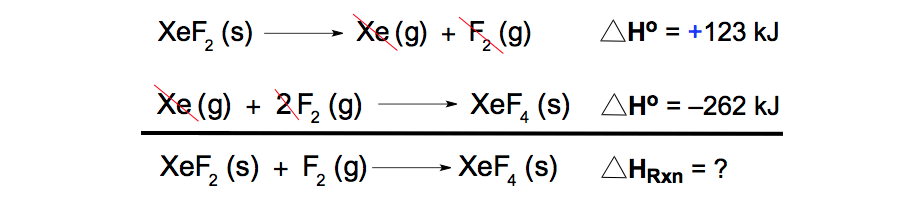Canceling Reaction Intermediates

STEP 5: Canceling out the intermediates gives us the overall chemical equation and by adding the two ΔHo values of the thermochemical steps you can isolate the ΔHRxnCalculation of Enthalpy of Reaction

Now test your skills with a more complex Hess’s Law problem.

PRACTICE 2: Find the ΔHRxn for the given overall chemical reaction:Thermochemical Equation (Carbon Dioxide)

The given thermochemical steps and ΔH values are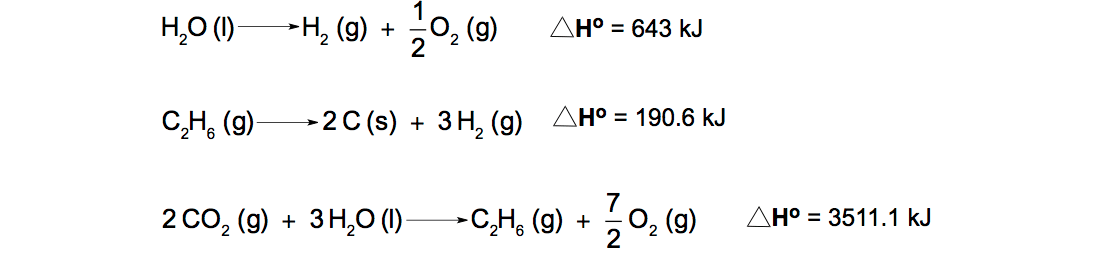Thermochemical Steps (Carbon Dioxide)

STEP 1: Start with CO­2 from the overall chemical equation and locate where it is in the given thermochemical steps. If CO2 does not match the CO in the overall chemical equation then we will need to manipulate the thermochemical step.Thermochemical Step (Carbon Dioxide)In our thermochemical step CO2 is listed as a reactant like we need, but there are two moles of it. In the overall chemical equation we have only 1 mole of COso we must divide the thermochemical equation by 2.Reversal of a Thermochemical Step

Realize that dividing the thermochemical step by 2 also means you divide your original ΔH value of 3511.1 kJ by 2. Dividing 3511.1 kJ by 2 gives a new ΔH value of 1755.55 kJ.

STEP 2: Continue to the next compound in the overall chemical equation, C (s), and locate where it is in the given thermochemical steps. If necessary, manipulate the thermochemical step.Thermochemical Step (Carbon solid)

In our thermochemical step we have two moles of C (s) listed products, but we need only 1 mole. So we divide the thermochemical step by 2.Thermochemical Step (Dividing by 2)

Dividing by 2 means we also divide the ΔH value by 2.

STEP 3: Next we search for O2 in the given thermochemical steps. If necessary, manipulate the thermochemical step.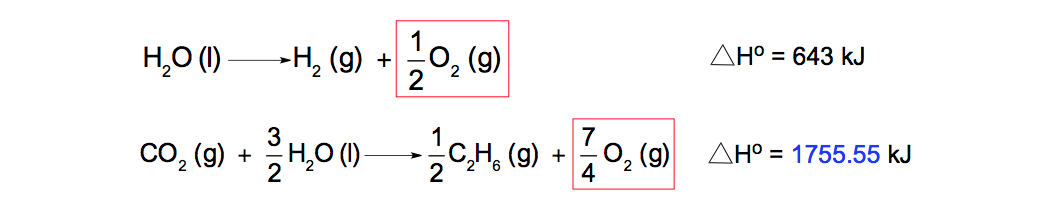Thermochemical Steps (Oxygen Gas)We find O2 in more than one thermochemical step so we can ignore it for it.

STEP 4: Now bring down all the thermochemical steps and cancel out the reaction intermediates to obtain the overall chemical equation.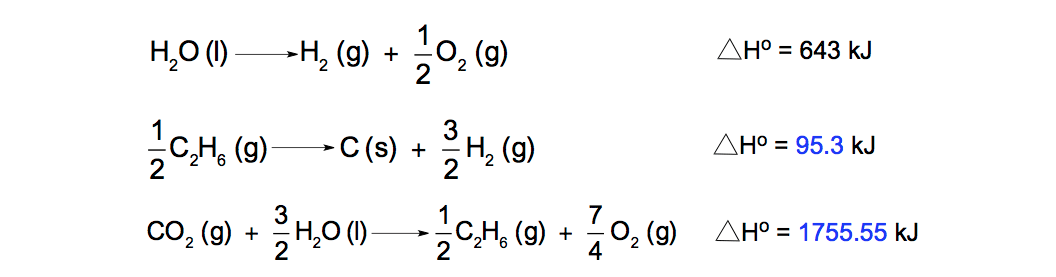Thermochemical Steps (Carbon Dioxide)We must cancel out H2O and H2 because they are not found in the overall chemical equation. In order to cancel out the H2 and H­2O molecules we will need to reverse and multiple the first thermochemical step by 1.5 or 3/2.Thermochemical Step (Reversal & Multiplication)

Reversing the thermochemical step reverses the sign for the ΔH value and multiplying the thermochemical step by 1.5, which is the same as 3/2, means we multiply the ΔH value by 1.5. Now we bring down all the thermochemical steps again and finally cancel out all reaction intermediates.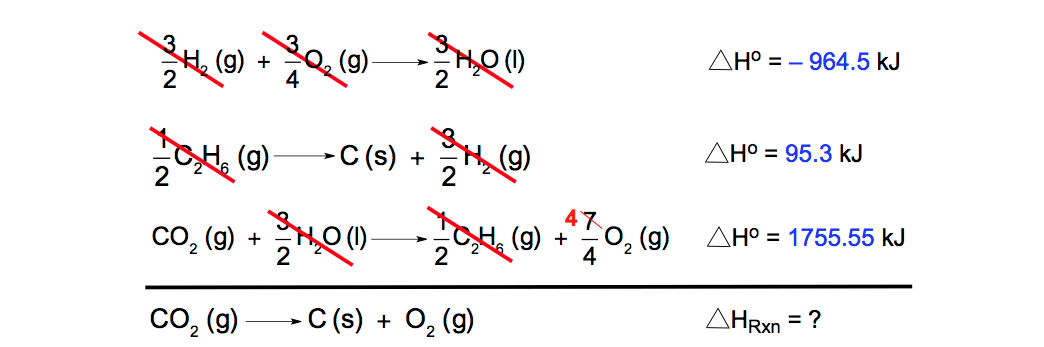Canceling Reaction Intermediates (Carbon Dioxide)

STEP 5: Canceling out the intermediates gives us the overall chemical equation and by adding the three ΔHo values of the thermochemical steps you can isolate the ΔHRxnOverall Enthalpy Change

Hess’s Law serves as one component in our understanding of the branch of chemistry dealing with thermal and chemical energy called Thermochemistry. Under Thermochemistry, we examine the concepts of calorimetry, the conservation of energy, internal energyformation equations, thermochemical equations and stoichiometryJules Bruno

Jules felt a void in his life after his English degree from Duke, so he started tutoring in 2007 and got a B.S. in Chemistry from FIU. He’s exceptionally skilled at making concepts dead simple and helping students in covalent bonds of knowledge.